GATE  >  Test: Spanning Tree

# Test: Spanning Tree

Test Description

## 24 Questions MCQ Test GATE Computer Science Engineering(CSE) 2023 Mock Test Series | Test: Spanning Tree

Test: Spanning Tree for GATE 2022 is part of GATE Computer Science Engineering(CSE) 2023 Mock Test Series preparation. The Test: Spanning Tree questions and answers have been prepared according to the GATE exam syllabus.The Test: Spanning Tree MCQs are made for GATE 2022 Exam. Find important definitions, questions, notes, meanings, examples, exercises, MCQs and online tests for Test: Spanning Tree below.
Solutions of Test: Spanning Tree questions in English are available as part of our GATE Computer Science Engineering(CSE) 2023 Mock Test Series for GATE & Test: Spanning Tree solutions in Hindi for GATE Computer Science Engineering(CSE) 2023 Mock Test Series course. Download more important topics, notes, lectures and mock test series for GATE Exam by signing up for free. Attempt Test: Spanning Tree | 24 questions in 90 minutes | Mock test for GATE preparation | Free important questions MCQ to study GATE Computer Science Engineering(CSE) 2023 Mock Test Series for GATE Exam | Download free PDF with solutions
 1 Crore+ students have signed up on EduRev. Have you?
Test: Spanning Tree - Question 1

### Let G be a weighted connected undirected graph with distinct positive edge weights. If every edge weight is increased by the same value, then which of the following statements is/are TRUE? P: Minimum spanning tree of  does not change. Q: Shortest path between any pair of vertices does not change.

Detailed Solution for Test: Spanning Tree - Question 1

Statement P is true.
For statement Q consider a simple graph with 3 nodes.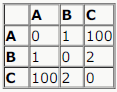Shortest path from A to C is A-B-C = 1 + 2 = 3
Now if the value of each edge is increased by 100,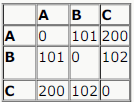The shortest path from A to C is A-C = 200, (A-B-C = 101 + 102 = 203)

Test: Spanning Tree - Question 2

###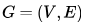is an undirected simple graph in which each edge has a distinct weight, and e is a particular edge of G . Whichof the following statements about the minimum spanning trees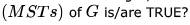I. If e is the lightest edge of some cycle in G, then every MST of G includes . II. If e is the heaviest edge of some cycle in G, then every MST of G excludes e .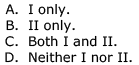Detailed Solution for Test: Spanning Tree - Question 2

I think answer is option B
Statement 2 is correct absolutely. if e is the heaviest edge in cycle every mst excludes it. Regarding statement 1, It is not fully right i think. When we think of a complete graph with 4 vertices and edge weights 1,2,5,6 in non diagonal and diagonal edges 3 and 4. 4,5,6 will create a cycle and we can exclude the lighest edge e (4)
from it, in a MST

So i think answer could be B

*Multiple options can be correct
Test: Spanning Tree - Question 3

### Choose the correct alternatives (more than one may be correct) and write the corresponding letters only: Kruskal’s algorithm for finding a minimum spanning tree of a weighted graph G with n vertices and  edges has the time complexity of:

Detailed Solution for Test: Spanning Tree - Question 3

When Union-Find algorithm is used to detect cycle while constructing the MST time complexity is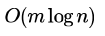where m is the number of edges, and n is the number of vertices. Since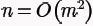in a graph, options B and E are also correct as
big-O specifies asymptotic upper bound only.

Ref: http://www.geeksforgeeks.org/greedy-algorithms-set-2-kruskals-minimum-spanning-tree-mst/

Test: Spanning Tree - Question 4

Let G be an undirected connected graph with distinct edge weights. Let emax be the edge with maximum weight and the edge with minimum weight. Which of the following statements is false?

Detailed Solution for Test: Spanning Tree - Question 4

In kruskal’s algorithm, we pick the edges in ascending order and add them to the forest if no cycle is formed. Option A is True because first edge could never create a cycle.
The only reason for emax to be present in the minimum spanning tree could be that it is the only possible edge to cover a particular vertex in a tree since all vertices have to be present in a spanning tree by definition.

Test: Spanning Tree - Question 5

Let G be a weighted undirected graph and e be an edge with maximum weight in G. Suppose there is a minimum weight spanning tree in G containing the edge e. Which of the following statements is always TRUE?

Detailed Solution for Test: Spanning Tree - Question 5

Option a is always true
Option b is not true when e is not part of a cycle.
Option c is not true when e is part of a cycle and all edge weights are same in that cycle
Option d is need not be true when e is not part of a cycle
Option a is always true as only the min weight edge in a cut set will be part of a minimum spanning tree.

Test: Spanning Tree - Question 6

Consider a weighted complete graph G on the vertex set {v1,v2,.....vn} such that the weight of the edge (vi, vj)  is . The weight of a minimum spanning tree of G is:

Detailed Solution for Test: Spanning Tree - Question 6

2(n-1) the spanning tree will traverse adjacent edges since they contain the least weight.

Test: Spanning Tree - Question 7

Consider a complete undirected graph with vertex set {0, 1, 2, 3, 4}. Entry Wij in the matrix W below is the weight of the edge {i, j}.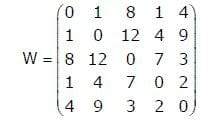In the graph given in above question, what is the minimum possible weight of a path P from vertex 1 to vertex 2 in this graph such that P contains at most 3 edges?

Detailed Solution for Test: Spanning Tree - Question 7

Path: 1 -> 0 -> 4 -> 2
Weight: 1 + 4 + 3

Test: Spanning Tree - Question 8

Let ω be the minimum weight among all edge weights in an undirected connected graph. Let e be a specific edge of weight ω . Which of the following is FALSE?

Detailed Solution for Test: Spanning Tree - Question 8

D is the false statement.

A minimum spanning tree must have the edge with the smallest weight (In Kruskal's algorithm we start from the smallest weight edge). So, C is TRUE.

If e is not part of a minimum spanning tree, then all edges which are part of a cycle with e, must have weight ≤ e, as otherwise we can interchange that edge with e and get another minimum spanning tree of lower weight. So, B and A are also TRUE.

Test: Spanning Tree - Question 9

For the undirected, weighted graph given below, which of the following sequences of edges represents a correct execution of Prim's algorithm to construct a Minimum Spanning Tree?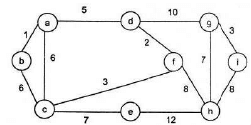Detailed Solution for Test: Spanning Tree - Question 9

Prim's algorithm starts with from any vertex and expands the MST by adding one vertex in each step which is close to the Intermediate MST(made till previous step).

Therefore correct answer would be (C).

(A): (d,f) is chosen but neither d nor f vertices are part of the previous MST(MST made till previous step).

(B): (g,h) is chosen but neither g or h vertices are part of the previous MST(MST made till previous step).

(D): (f,c) is chosen but at that point (f,d) is close to the intermediate MST.

Test: Spanning Tree - Question 10

Consider the following graph: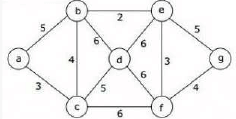Which one of the following is NOT the sequence of edges added to the minimum spanning tree using Kruskal’s algorithm?

Detailed Solution for Test: Spanning Tree - Question 10

in option d b-c with weight 4 is added before a-c with weight 3 is added. In kruskal's algorithm edges should be added in non decreasing order of weight
So option D may be correct

Test: Spanning Tree - Question 11

Consider a complete undirected graph with vertex set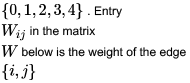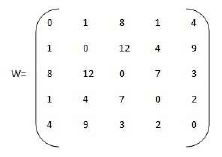What is the minimum possible weight of a spanning tree T in this graph such that vertex 0 is a leaf node in the tree T?

Detailed Solution for Test: Spanning Tree - Question 11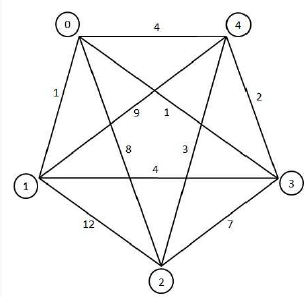Answer is (D) 10. The edges of the spanning tree are: 0 - 1, 1 - 3, 3 - 4, 4 - 2. Total Weight = 10

Test: Spanning Tree - Question 12

An undirected graph  G (V,E) contains n(n>2) nodes named v1,v2,.......vn. Two nodes  vi,vj are connected if and only if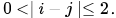Each edge  (vi,vj) is assigned a weight i=+j. A sample graph with n=4 is shown below.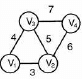What will be the cost of the minimum spanning tree (MST) of such a graph with n nodes?

Detailed Solution for Test: Spanning Tree - Question 12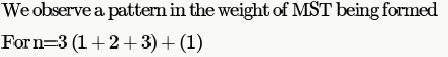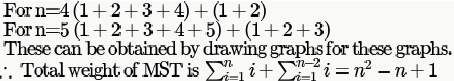Test: Spanning Tree - Question 13

Consider a complete undirected graph with vertex set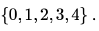Entry  Wij in the matrix  W below is the weight of the edge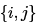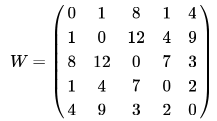What is the minimum possible weight of a path P from vertex 1 to vertex 2 in this graph such that P contains at most 3 edges?

Detailed Solution for Test: Spanning Tree - Question 13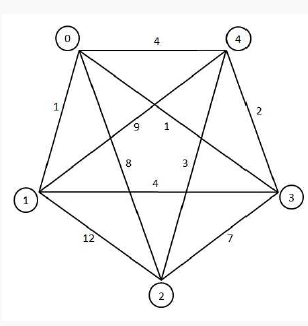Answer is (B) 8. The possible path is: 1 - 0, 0 - 4, 4 - 2.

Test: Spanning Tree - Question 14

An undirected graph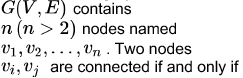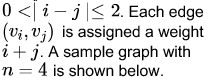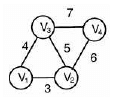The length of the path from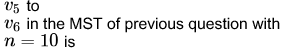Detailed Solution for Test: Spanning Tree - Question 14

There is no direct edge between Vi and Vi+1 vertex in mst except V1 to V2 so path from Vi and Vi+1 includes all edges from v1 to Vi+1 which are in mst:

edge weights in mst:

=3 + 4 + 6 + 8 + 10 + 12 +...+*(2(n-1))

=1+(2+ 4+6+....till n-1 terms)
=1+ 2(1+2+3+4+...n-1)

=1+(n-1)*n=n2-n+1
In this case v6 = 62-6+1 =31

Test: Spanning Tree - Question 15

Let G be a weighted graph with edge weights greater than one and G' be the graph constructed by squaring the weights of edges in G. Let T and T' be the minimum spanning trees of G and G', respectively, with total weights t and t'. Which of the following statements is TRUE?

Detailed Solution for Test: Spanning Tree - Question 15

When the edge weights are squared the minimum spanning tree won't change.

t'< tbecause sum of squares is always less than the square of the sums except for a single element case.

Hence, B is the general answer and A is also true for a single edge graph.

Test: Spanning Tree - Question 16

Let G be a connected simple graph (no self-loops or parallel edges) on n ≥ 3vertices, with distinct edge weights. Let e1,e2........em be an ordering of the edges in decreasing order of weight. Which of the following statements is FALSE?

Detailed Solution for Test: Spanning Tree - Question 16

a) & c) are trivially true. Edge with max value e1 must be present in Maximum spanning tree & same with minimum.
e) This is true, because all egde weights are distinct. maximum spanning tree is unique.
b) e1 & e2 must be present in Maximum spanning tree. I'll prove it using Kruskal Algorithm.

We will first insert weight with biggest value, e1. Then we insert e2 (second highest) . 2 edges do not create cycle. Then
we can go on from there inserting edges according to edge weights.As they have just asked for top 2 edges, using Kruskal
Algo we can say that top 2 edges must be in Maximum spanning tree.

d)
This is false. There are chances that this em weight edge is cut edge(Bridge) Then it must be inserted to from any spanning tree.

We can not say the same for Top 3 as they can create cycle & They we can not take a3 to make spanning tree.

Test: Spanning Tree - Question 17

In a connected weighted graph with  vertices, all the edges have distinct positive integer weights. Then, the maximum number of minimum weight spanning trees in the graph is

Detailed Solution for Test: Spanning Tree - Question 17

There will be unique min weight spanning tree since all weights are distinct.

Test: Spanning Tree - Question 18

Consider the following undirected connected graph G with weights on its edges as given in the figure below. A minimum spanning tree is a spanning tree of least weight and a maximum spanning tree is one with largest weight. A second best minimum spanning tree whose weight is the smallest among all spanning trees that are not minimum spanning trees in G.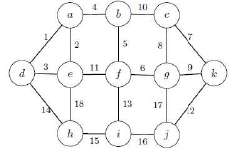Which of the following statements is TRUE in the above graph? (Note that all the edge weights are distinct in the above graph)

Detailed Solution for Test: Spanning Tree - Question 18

In the graph we have all edge weights are distinct so we will get unique minimum and maximum spanning tree.

Each Cycle must exclude maximum weight edge in minimum spanning tree.
Here we have two cycle of 3 edges , ade and cgk .
for second best minimum spanning tree = exclude ae edge and include de edge

other way : second best minimum spanning tree= exclude cg edge and include gk edge.
so e should be the ans.

*Answer can only contain numeric values
Test: Spanning Tree - Question 19

Let  be a connected undirected graph of 100 vertices and 300 edges. The weight of a minimum spanning tree of  is 500. When the weight of each edge of  is increased by five, the weight of a minimum spanning tree becomes ______.

Detailed Solution for Test: Spanning Tree - Question 19

first find no of edges in mst...
mst has n-1 edges where n is no of vertices. 100-1 =99 edges
each 99 edges in mst increases by 5 so weight in mst increased 99*5=495
now total weight of mst =500+495=995

*Answer can only contain numeric values
Test: Spanning Tree - Question 20

The graph shown below has 8 edges with distinct integer edge weights. The minimum spanning tree (MST) is of weight 36 and contains the edges: {(A, C), (B, C), (B, E), (E, F), (D, F)}. The edge weights of only those edges which are in the MST are given in the figure shown below. The minimum possible sum of weights of all 8 edges of this graph is_______________.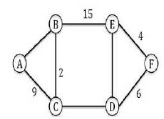Detailed Solution for Test: Spanning Tree - Question 20

Consider the cycle ABC. AC and AB are part of minimum spanning tree. So, AB should be greater than max(AC, BC) (greater and not equal as edge weights are given to be distinct), as otherwise we could add AB to the minimum spanning tree and removed the greater of AC, BC and we could have got another minimum spanning tree. So, AB > 9.

Similarly, for the cycle DEF, ED > 6.
And for the cycle BCDE, CD > 15.

So, minimum possible sum of these will be 10 + 7 + 16 = 33. Adding the weight of spanning tree, we get the total sum of
edge weights

= 33 + 36 = 69

*Answer can only contain numeric values
Test: Spanning Tree - Question 21

The number of distinct minimum spanning trees for the weighted graph below is _____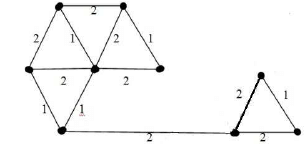*Answer can only contain numeric values
Test: Spanning Tree - Question 22

How many minimum spanning trees does the following graph have? Draw them. (Weights are assigned to edges).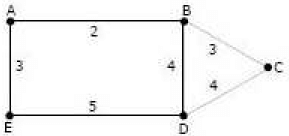Detailed Solution for Test: Spanning Tree - Question 22

2 only.
{AB,BC,AE,BD} and {AB,BC,AE,CD}.

Test: Spanning Tree - Question 23

An undirected graph G(V, E) contains n ( n > 2 ) nodes named v1 , v2 ,….vn. Two nodes v, vj are connected if and only if 0 < |i – j| <= 2. Each edge (vi, vj ) is assigned a weight i + j. A sample graph with n = 4 is shown below.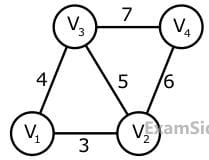The length of the path from v5 to v6 in the MST of previous question with n = 10 is

*Answer can only contain numeric values
Test: Spanning Tree - Question 24

Let G be a complete undirected graph on 4 vertices, having 6 edges with weights being 1, 2, 3,  4, 5, and 6. The maximum possible weight that a minimum weight spanning tree of G can have is __________

Detailed Solution for Test: Spanning Tree - Question 24

it is said maximum weight pssbl.

draw a triangle. 3 sides weight 1 2 3. and 4th point is in center. join it with tringle vertices.. got more 3 sides. new side weight 4 5 6. now draw mst. take 1 take 2. cant take 3, so take 4. 1+2+4=7

## GATE Computer Science Engineering(CSE) 2023 Mock Test Series

136 docs|165 tests
 Use Code STAYHOME200 and get INR 200 additional OFF Use Coupon Code
Information about Test: Spanning Tree Page
In this test you can find the Exam questions for Test: Spanning Tree solved & explained in the simplest way possible. Besides giving Questions and answers for Test: Spanning Tree, EduRev gives you an ample number of Online tests for practice

## GATE Computer Science Engineering(CSE) 2023 Mock Test Series

136 docs|165 tests